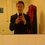# No need for compound angle formulae.....

Usually when you need to calculate trigonometry values such as sin15 and cos15 then you can use: $sin(\theta\pm \phi) = sin(\theta)cos(\phi)\pm sin(\phi)cos(\theta)$ However, I found an alternative method using geometry directly.

Firstly you draw an isosceles triangle ABC (A is the top angle) with A = 30, B=C = 75. Now draw a line from B that is perpendicular to AC at a point D. This means that $\angle$ABD = 60 and $\angle$DBC = 15 $\Rightarrow$ $\angle$BCD = 75. Also, without loss of generality, let AB = 2

By using special triangle AB = 2, BD = 1 and AD = $\sqrt{3}$ $\Rightarrow$ DC = 2 - $\sqrt{3}$. Now by using pythagoras' theorem: $BC = \sqrt{1 + (2-\sqrt{3})^2 } = \sqrt{8-4\sqrt{3}} = \sqrt{(\sqrt{6} - \sqrt{2})^2} = \sqrt{6} - \sqrt{2}$ So now we have a rightangle triangle BCD where all the sides are known.

Finally; $cos75 = sin15 = \frac{opposite}{Hypotenuse}\ = \frac{2-\sqrt{3}}{\sqrt{6} - \sqrt{2}}\times\frac{\sqrt{6} + \sqrt{2}}{\sqrt{6} + \sqrt{2}} = \frac{\sqrt{6} - \sqrt{2}}{4}$ $sin75 = cos15 = \frac{1}{\sqrt{6} - \sqrt{2}}\ = \frac{\sqrt{6} + \sqrt{2}}{4}$ $tan15 = \frac{opposite}{adjacent} = 2 - \sqrt{3} \ and \ tan75 = \frac{1}{2-\sqrt{3}} = 2+\sqrt{3}$Note by Curtis Clement
6 years, 4 months ago

This discussion board is a place to discuss our Daily Challenges and the math and science related to those challenges. Explanations are more than just a solution — they should explain the steps and thinking strategies that you used to obtain the solution. Comments should further the discussion of math and science.

When posting on Brilliant:

• Use the emojis to react to an explanation, whether you're congratulating a job well done , or just really confused .
• Ask specific questions about the challenge or the steps in somebody's explanation. Well-posed questions can add a lot to the discussion, but posting "I don't understand!" doesn't help anyone.
• Try to contribute something new to the discussion, whether it is an extension, generalization or other idea related to the challenge.
• Stay on topic — we're all here to learn more about math and science, not to hear about your favorite get-rich-quick scheme or current world events.

MarkdownAppears as
*italics* or _italics_ italics
**bold** or __bold__ bold
- bulleted- list
• bulleted
• list
1. numbered2. list
1. numbered
2. list
Note: you must add a full line of space before and after lists for them to show up correctly
paragraph 1paragraph 2

paragraph 1

paragraph 2

[example link](https://brilliant.org)example link
> This is a quote
This is a quote
    # I indented these lines
# 4 spaces, and now they show
# up as a code block.

print "hello world"
# I indented these lines
# 4 spaces, and now they show
# up as a code block.

print "hello world"
MathAppears as
Remember to wrap math in $$ ... $$ or $ ... $ to ensure proper formatting.
2 \times 3 $2 \times 3$
2^{34} $2^{34}$
a_{i-1} $a_{i-1}$
\frac{2}{3} $\frac{2}{3}$
\sqrt{2} $\sqrt{2}$
\sum_{i=1}^3 $\sum_{i=1}^3$
\sin \theta $\sin \theta$
\boxed{123} $\boxed{123}$

## Comments

Sort by:

Top Newest

Nice work !!!!!

- 6 years, 4 months ago

Log in to reply

Thankyou :)

- 6 years, 4 months ago

Log in to reply

×

Problem Loading...

Note Loading...

Set Loading...